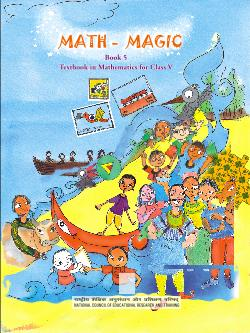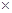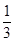# NCERT Solutions for Class 5 Maths The Fish Tale## myCBSEguide App

CBSE, NCERT, JEE Main, NEET-UG, NDA, Exam Papers, Question Bank, NCERT Solutions, Exemplars, Revision Notes, Free Videos, MCQ Tests & more.

NCERT solutions for Class 5 Mathematics## NCERT Solutions for Class 5 Maths The Fish Tale

###### (1) At what price per kg did Fazila sell the kingfish?

Sol (1) Price of 8kg kingfish=Rs.1200

Price of 1kg kingfish=Rs. (1200/8)

= Rs. 150

NCERT Solutions for Class 5 Maths The Fish Tale

###### (2) Floramma has sold 10kg prawns today. How much money did she get for that?

Sol. (2) Price of 1kg prawns= Rs. 150

Price of 10kg prawns=Rs. (10150)

=Rs. 1500

###### (3) Gracy sold 6 kg sword fish. Mini has earned as much money as Gracy. How many kg of sardines did Mini sell?

Sol. (3) Cost of 1 kg swordfish= Rs. 60

Gracy earned on selling 6 kg swordfish=Rs. (660) = Rs. 360

Earning of mini = Rs. 360

Cost of Sadines=Rs. 40 per kg

Quantity of sardines sold for Rs. 360= (360/40) kg= 9kg

###### (4) Basher has Rs.100. He spends one-fourth of the money on squid and another three-fourth on prawns.

(a) How many kilograms of squid did he buy?

(b) How many kilograms of prawns did he buy?

Sol. We have,

One-fourth of Rs.100 =Rs. (100/4) =Rs.25

Three-fourth of Rs.100= Rs. (100/4)3 = Rs. (253) = Rs.75

(a) Cost of Squid= Rs. 50 per kg.

For Rs. 25, quantity of squid brought= half kg

[Half of Rs. 50 is Rs. 50]

(b) Cost of prawns= Rs. 150 per kg

For Rs. 75, quantity of prawns brought= half kg

[Half of Rs. 150 is Rs. 150]

NCERT Solutions for Class 5 Maths The Fish Tale

###### Ques. Women’s ‘Meenkar Bank’

The meeting of the Meenkar Bank has just begun. Fazila is the president. Twenty fisherwomen have made their own bank. Each saves Rs. 25 every month and puts it in the bank.

1.How much money does the group collect each month?

2. How much money will be collected in ten years?

Sol. (1) Money collected by each member is Rs. 25 per month.

Money collected by the group of 20 members per month=Rs. (2025) =Rs. 500

(2) Money collected by the group in 10 years= Rs. (1012500) =Rs. 60000

NCERT Solutions for Class 5 Maths The Fish Tale

###### Ques. (a) Gracy took a loan of Rs. 4000 to buy a net. She paid back Rs. 345 every month for one year. How much money did she pay back to the bank?

Sol. (a) Loan taken by Gracy=Rs. 4000

Amount paid at the rate of Rs. 345 per month for one year= Rs. (34512) =Rs. 4140

Gracy paid back to the bank Rs. 4140

(b) Jhansi and her sister took a loan of Rs. 21000 to buy a long boat. They paid back a total of Rs. 23520 in one year. How much did they pay back every month?

Sol. (b) Amount paid back by Jhansi and her sister to the bank in one year i.e. 12months =Rs. 23250

Amount paid back every month=Rs. (23250/12) =Rs. 1960

NCERT Solutions for Class 5 Maths The Fish Tale

###### Ques. Why Don’t We Start a New Fish-drying Factory?

The women of Meenkar Bank also want to start a factory to dry fish. The Panchayat has given them some land for that. Over the years they have saved Rs 74,000. They find out how much they will need for the factory. Fazila writes the things they need to buy to begin. See the table for the cost of each item and the number of items they want to buy. Find the total cost.

 Item Price of each No. of items Cost Borewell for fresh water Rs. 3000 1 Bamboo for fish drying Rs. 2000 20 Cement tank Rs. 1000 4 Tray and knife Rs. 300 20 Bucket Rs. 75 20

Total cost to set up the factory = ____________

When fresh fish is dried it becomesits weight. In one month they plan to dry 6000 kg of fresh fish. How much dried fish will they get in a month? ____________

Sol.

 Item Price of each No. of items Cost Borewell for fresh water Rs. 3000 1 Rs. (30001) =Rs. 3000 Bamboo for fish drying Rs. 2000 20 Rs. (200020) = Rs. 40000 Cement tank Rs. 1000 4 Rs. (10004) =Rs. 4000 Tray and knife Rs. 300 20 Rs. (30020) = Rs. 6000 Bucket Rs. 75 20 Rs. (7520) =Rs. 1500

Total cost to set up the factory = Rs. 54500

Quantity of dried fish obtained in one month=of 6000 kg = (6000/3) kg= 2000kg

###### Ques. Let us first calculate for 6 kg of fresh fish.

We buy fresh fish for Rs. 15 per kg

We sell dried fish for Rs. 70 per kg

We dry 6 kg fresh fish to get ………. Kg dried fish

For 6 kg fresh fish we have to pay 6………. = Rs. 90

We will sell 2 kg dried fish and get 2………. = Rs.………

So if we dry 6 kg fresh fish we will earn ………-90= Rs. ……….

But it we dry 6000 kg we can earn Rs…………1000 in one month!

Sol.  We dry 6 kg fresh fish to get 2kg dried fish [6/3=2]

For 6 kg fresh fish we have to pay 6Rs. 15 =Rs. 90

We will sell 2kg dried fish and get 2Rs. 70 =Rs. 140

So if we dry 6 kg fresh fish we will earn Rs. 140-Rs. 90= Rs. 50

But if we dry 6000 kg we can earn Rs 501000=Rs. 5000 in one month!

NCERT Solutions for Class 5 Maths The Fish Tale

###### Ques. Jhansi- I found that for 6000 kg fish we would need 1500 kg salt every month! Its price is Rs. 2 per kg

Monthly costs:

(a) Salt 15002= Rs.………..

(b) Packing and bus charges= Rs. 3000

So the total monthly cost of drying and selling the fish =Rs. ………..

Fazila- That sounds very good! Our calculations tell us that every month our Bank will earn Rs. 44000!

1. Check to see if you also get the same answer.

Sol.  Monthly costs-

(a) Salt =1500Rs. 2= Rs. 30000

(b) Packing and bus charges= Rs. 3000

So, the total monthly cost of drying and selling the fish=Rs. 3000+Rs. 3000= Rs. 6000

They earn Rs. (50000-6000) = Rs. 44000 per month

## NCERT Solutions for Class 5 Mathematics Chapter 1 The Fish Tale

NCERT Solutions Class 5 Maths PDF (Download) Free from myCBSEguide app and myCBSEguide website. Ncert solution class 5 Maths includes text book solutions from Class 5 Maths Book . NCERT Solutions for CBSE Class 5 Maths have total 14 chapters. 5 Maths NCERT Solutions in PDF for free Download on our website. Ncert Maths class 5 solutions PDF and Maths ncert class 5 PDF solutions with latest modifications and as per the latest CBSE syllabus are only available in myCBSEguide.

## CBSE app for Students

To download NCERT Solutions for Class 5 Maths, Social Science Computer Science, Home Science, Hindi English, Maths Science do check myCBSEguide app or website. myCBSEguide provides sample papers with solution, test papers for chapter-wise practice, NCERT solutions, NCERT Exemplar solutions, quick revision notes for ready reference, CBSE guess papers and CBSE important question papers. Sample Paper all are made available through the best app for CBSE students and myCBSEguide website.Test Generator

Create question papers online with solution using our databank of 5,00,000+ questions and download as PDF with your own name & logo in minutes.

### 4 thoughts on “NCERT Solutions for Class 5 Maths The Fish Tale”

1. Nice

2. Mujhe to maje a gaye sare answer copy kar lie oye hoe

3. It helps very much in teaching n giving practice to my daughter

4. It is very nice